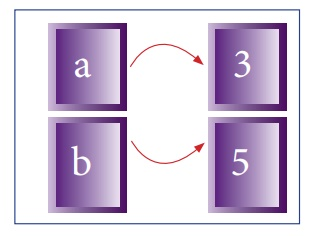Home | | Computer Science 12th Std | Variable Scope

# Variable Scope

To understand the scope of variables in a programming language, it is important to learn about what variables really are.

Variable Scope

To understand the scope of variables in a programming language, it is important to learn about what variables really are. Essentially, they're addresses (references, or pointers), to an object in memory. When you assign a variable with := to an instance (object), you're binding (or mapping) the variable to that instance. Multiple variables can be mapped to the same instance.

Note

The process of binding a variable name with an object is called mapping. = (equal to sign) is used in programming languages to map the variable and object.

Programming languages keeps track of all these mappings with namespaces. Namespaces are containers for mapping names of variables to objects. You can think of them as dictionaries, containing list of words and its meanings. The words are mapped with its meaning in dictionaries whereas names are mapped with objects (name : = object) in programming language. This allows access to objects by names you choose to assign to them.

In the following example, a is first mapped to the integer 5. In this case, a is the variable name, while the integer value 5 is the object.

Then, b is set equal to a. This actually means that b is now bound to the same integer value as a, which is 5.

1. a:=5

2. b:=aNow consider the following extension of the above statements

1. a:=5

2. b:=a

3. a:=3

If you then change a to be equal to 3, a budding programmer might expect b also be equal to 3, but that is not the case. b is still mapped (or pointing) to the integer value of 5. The only thing that changed is a, which is now mapped to the integer value 3.

Mapping after changing the value of aThe scope of a variable is that part of the code where it is visible. Actually, to refer to it, you don’t need to use any prefixes then. Let’s take an example,

1. Disp():

2. a:=7

When you try to display the value of a outside the procedure the program flags the error “name ‘a’ is not defined”. This is because the lifetime of the variable is only till the end of the procedure. Also, the duration for which a variable is alive is called its ‘life time’.

Study Material, Lecturing Notes, Assignment, Reference, Wiki description explanation, brief detail
12th Computer Science : Chapter 3 : Scoping : Variable Scope |